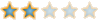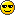Montreal Math ClubMathematics students in Montreal - Étudiants de mathématiques à MontréalHomeCalendarFAQSearchMemberlistUsergroupsRegisterLog in

 Montreal Math Club :: Mathematics :: Combinatorics Share

ProjectivityAuthorMessage

EuclidPosts : 95
Join date : 2009-09-15
Age : 57
Location : AlexandriaSubject: ProjectivityTue Sep 20, 2011 6:21 am How to generalize the Fano" = Klein equation ?there is a transitivity hierarchy (stick-n-stuck-all structures)1....... groups, affine spaces with translations1+ ... affine spaces with translations and dilatations2...... lines, fields3...... projective lines3+.... also projective lines 4,5.... the sporadic Mathiew groups.After groups and fields, the next symmetric objects are the projective lines.Reading from the Conway-Hulpke-Mckay table :9T27 - sharply triple transitive, the natural projective line with 9 pointsand 9T32 - triple transitive, but also a projective group PgamaL(2,.The extra-transitivity (more than three) of a projective line is explained by some twists of the line. Let' s say that the line is coordinated with some elements of a field; then, the automorphisms of that field will permute also the points on the line.http://en.wikipedia.org/wiki/CollineationThe nice case is p prime, whenProjectiveLine(p prime)'''= Lin (stick three stuck all)for p^k +1 points on the projective line,ProjectiveLine(p^k)''' = Cyc[k](Lin). (stick three and reach the twisting)that Gal(K/k) is cyclic of order k An affine line should be also twisted by field automorphisms, for example9T15 - the affine line on 9 points, sharply 2-transitive (order 72)and6T19 - the affine line with twists (order 144)EuclidPosts : 95
Join date : 2009-09-15
Age : 57
Location : AlexandriaSubject: Re: ProjectivityTue Sep 20, 2011 6:51 am The lines of a projective plane should be projective lines (at least this is the intention of every author).Then a nice projective plane, for p prime, having p^2+p+1 points, is described byProjectivePlane" = Cyc[p-1] . AffinePlane after pinning two points in a projective plane, a line is sketched. The remaining relabeling liberty comes from - the rest of the projective line, and secondly from- the remaining affine plane (without rotations) - after sticking one line, that could be considered the "infinite line", and thus blocking the directionsProjectivityPage 1 of 1

Permissions in this forum:You cannot reply to topics in this forumJump to: Select a forum||--Math Club|   |--Mathematical Sundays|   |--Suggestions|   |--Introduce yourself|   |--Mathematics|   |--Problem Solving|   |   |--Solved problems|   |   |   |--Calculus and Analysis|   |--Combinatorics|   |--Geometry|   |--Number Theory|   |--Probabilities and statistics|   |--Set theory and Topology|   |--Online Resources|   |--Chit chat    |--The Water Cooler    |--Mathematical poems